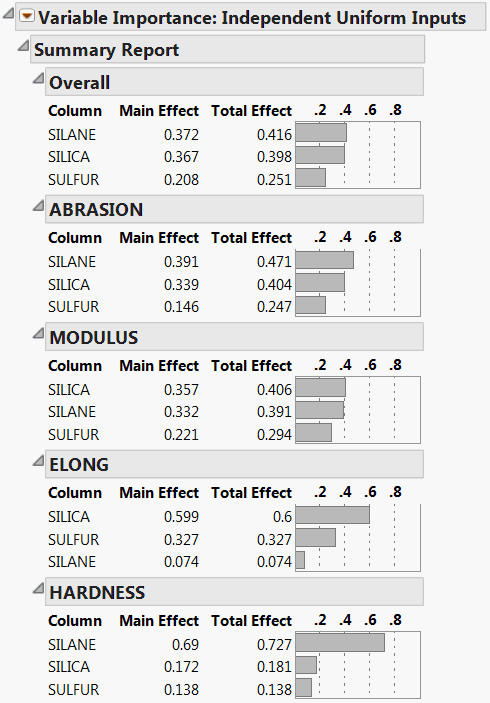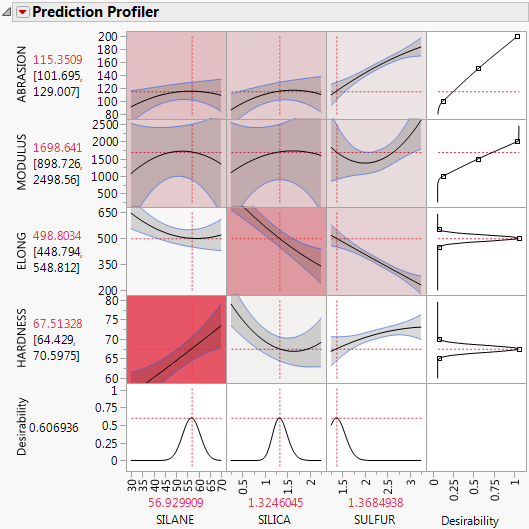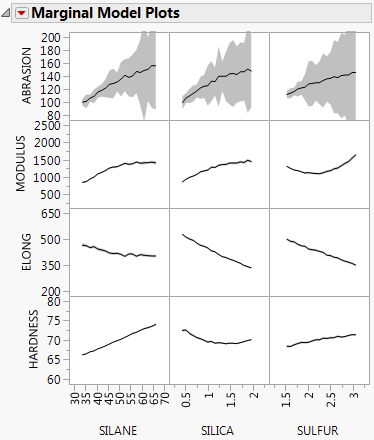Profilers > Profiler > Additional Examples of the Prediction Profiler > Example of Variable Importance for Multiple Responses

Publication date: 06/16/2020

## Example of Variable Importance for Multiple Responses

The data in the Tiretread.jmp sample data table are the result of a designed experiment where the factors are orthogonal. For this reason, you use importance estimates based on independent inputs. Suppose that you believe that, in practice, factor values vary throughout the design space, rather than assume only the settings defined in the experiment. Then you should choose Independent Uniform Inputs as the sampling scheme for your importance indices.

1. Select Help > Sample Data Library and open Tiretread.jmp.

2. Run the script RSM for 4 Responses.

The Prediction Profiler is displayed at the very bottom of the report.

3. Click the Prediction Profiler red triangle and select Assess Variable Importance > Independent Uniform Inputs.

The Summary Report is shown in Figure 3.28. Because the importance indices are based on random sampling, your estimates might differ slightly from those shown in the figure.

The report shows tables for each of the four responses. The Overall table averages the factor importance indices across responses. The factors in the Prediction Profiler (Figure 3.29) have been reordered to match their ordering on the Overall table’s Total Effect importance.

Figure 3.28 Summary Report for Four Responses4. Click the red triangle next to Variable Importance: Independent Uniform Inputs and select Colorize Profiler.

Colors from a red to white intensity scale are overlaid on profiler panels to reflect Total Effect importance. For example, you easily see that the most important effect is that of Silane on Hardness.

Figure 3.29 Prediction Profiler for Four ResponsesThe Marginal Model Plots report shows mean responses for each factor across a uniform distribution of settings for the other two factors.

Figure 3.30 Marginal Model Plots for Four Responses